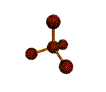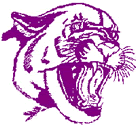##Mr. Rapp's Chemistry Help Site
 Grades  Graphing Tips  Online 3-D Laboratory  Reference Desk  AP Chemistry Test  News from Science  Links  After School Help  Fun Stuff  Teachers' Lounge    BHSS Chemistry  E-mail Mr. RappIntroduction to Chemistry and Measurements Students should be able to... Define chemistry Write the symbol and name of common elements Describe the major areas of study in chemistry (organic, inorganic, analytical, physical, and biochemistry) Describe the difference between mass and weight Describe the difference between accuracy and precision Describe the scientific method and how its used List the SI base units for length, mass, volume, time, and temperature List and convert using the SI prefixes: kilo-, centi-, and milli- Convert temperatures from degrees Celcius to Kelvin (add 273) or from Kelvin to degrees Celcius (subtract 273) Convert mass, length, and volume, and temperature between the SI and English systems (2.54 cm = 1 inch, 454 g = 1 pound, .946 L = 1 quart) Calculate the density of a solid (mass / volume) Express numbers in scientific notation Express scientific notation in decimal form Compute numbers in scientific notation using a calculator Be familiar with the following terms:  theory, law Decide if a digit in a number is "significant" Round answers to arithmetic problems to reflect the precision of the number Sample Problems for Chapters 1 and 2 Convert. 1. 58.2 mm to cm 2. 63.1 degrees C to Kelvin 3. 13.5 km to miles 4. 1.31 L to mL 5. 8750 seconds to hours 6. 13 gallons to L 7. 20000 seconds to days 8. 202 kilograms to pounds Express in scientific notation. 9. 45300000 ____________________________________ 10. 0.000461 ____________________________________ 11. 0.0212 ____________________________________ 12. 3430 ____________________________________ 13. 0.002 ____________________________________ Express in decimal form. 14. 4.94 X 103 __________________ 15. 2.312 X 10-5 __________________ 16. 1.11 X 103 __________________ 17. 3.92 X 103 __________________ 18. 8.72 X 10-2 __________________ State the number of significant digits in each measurement. 19. 470 birds _____ 20. 0.003 mL _____ 21. 320. g _____ 22. 0.2540 km _____ 23. 200000 ants _____ Solve. Round each answer accordingly. 24. 480 X 2.875 = 25. 9.81 / 4 = 26. 2.34 + 5.1204 + 9.63 = Convert. 27. 98 degrees C to Kelvin 28. 73 degrees C to K 29. 374 K to degrees C 30. 17 K to degrees C 31. Breifly describe the five main areas of chemistry.E-mail Mr. Rapp | BHSS Chemistry Web SiteLast updated on December 2, 2002 8:44 AM